A MODEL FOR THE SIMULATION OF MACROALGAL POPULATION DYNAMICS AND PRODUCTIVITY

P. Duarte and J.G. Ferreira

Dept. Environmental Sciences & Engineering, Faculty of Sciences and Technology

New University of Lisbon

2825 Monte de Caparica, Portugal

joao.imar@mail.telepac.pt

ABSTRACT

In this work a mathematical model to simulate the population dynamics and productivity of macroalgae is described. The model calculates the biomass variation of a population divided into size-classes. Biomass variation in each class is estimated from the mass balance of carbon fixation, carbon release and demographic processes such as mortality and frond breakage. The transitions between the different classes are calculated in biomass and density units as a function of algal growth. Growth is computed from biomass variations using an allometric relationship between weight and length. Gross and net primary productivity is calculated from biomass production and losses over the period of simulation. The model allows the simulation of different harvesting strategies of commercially important species. The cutting size and harvesting period may be changed in order to optimise the calculated yields.

In this study the model was used with the agarophyte Gelidium sesquipedale (Clem.) Born. et Thur.. This species was chosen because of its economic importance as a the main raw material for the agar industry. Net primary productivity calculated with it and from biomass variations over a yearly period, gave similar results. The results obtained suggest that biomass dynamics and productivity are more sensitive to the water light extinction coefficient than to the precision in biomass estimates, used as initial conditions for the model. Model results also suggest that biomass losses due to respiration and exudation are comparable to those resulting from mortality and frond breakage. During winter, a significant part of the simulated population has a negative net productivity. The importance of considering different parameters in the productivity light relationships in order to account for their seasonal variability is demonstrated with the model results.

The model was implemented following an object oriented programming approach. The programming methodology allows a fast adaptation of the model to other species without  major software development.

INTRODUCTION

Models to evaluate the population dynamics and productivity of macroalgae are not common in the literature, in spite of the importance of these algae in carbon fixation in coastal ecosystems. Models of macroalgal populations may be divided into two groups:

i - Demographic models, that simulate the numerical density of a population divided into size classes or different life history phases (e.g. Ang , 1987, 1990; Chapman, 1993; Santos, 1993a);

ii - Models that simulate the biomass density dynamics  (e.g. Brinkhuis, 1977; Seip et al., 1979; Seip, 1980a e b; Ferreira & Ramos, 1989; Duarte & Ferreira, 1993);

The first group is also known as "Leslie matrix models" due to the influence of this author's work in their development (Leslie, 1945, 1948). A revision on plant demographic models is given by Caswell (1986). The general matrix model form, that can be adapted for age, size or stage classified populations is, in matrix notation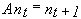(1)

Where nt is a column vector with elements nt,1, nt,2... representing the number of individuals in each class at time t and nt+1 is another column vector representing the number of individuals in each class at time t+1. A is the transition matrix containing the probability transitions between the classes.

These probabilities are a function of the growth, breakage, mortality and recruitment rates of the different classes. Growth is the net result of physiological and demographic time dependent processes leading to gains and losses of biomass. Transition probabilities may be assessed by tagging macroalgal fronds of different sizes and monitoring them during months or years, by measuring their length and counting the survivors between sampling times. The transition rates obtained are time-dependent due to various seasonal phenomena. This variability complicates the application of these models to forecasting, unless the transition probabilities are expressed as functions of the environmental variables on which they depend. However, usually this is not the case. An example of the application of this type of model to maximise harvest yields of marine macroalgae is found in Ang (1987).

In models of the second type, the population biomass is usually expressed as a whole (e.g. frequently as a carbon pool) without discriminating between size or life history phases. These models are often employed in primary productivity calculations. The biomass variations are usually computed from the physiological and demographic processes that are calculated as a function of physical and chemical parameters. The general equation for macroalgae is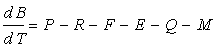(2)

Where,

B - Biomass, T - Time, P - Gross productivity, R - Respiratory rate, F - Photorespiratory rate, E - Exudation rate, Q - Frond breakage rate, M - Mortality.

During the last decades several mathematical formulations of algal primary productivity (P) as a function of light intensity have been developed (e.g. Steele, 1962; Jassby & Platt, 1976; Platt el al. 1980; Iwakuma & Yasuno, 1983, Eilers & Peeters, 1988). Equations that combine the effects of several environmental variables such as light intensity, temperature and nutrient concentrations can also be found (e.g. Keller, 1989). These mathematical formulations were developed mainly for phytoplankton although there seems to be no reason against their employment in macroalgal productivity, since the underlying physiological phenomenon is the same. It has been demonstrated that carbon fixation in marine macroalgae may also occur in the absence of light (Kremer, 1981). In some Phaeophyceae the dark carbon fixation may account for up to 50 % of the total carbon assimilated. In the Chlorophyceae and Rhodophyceae the dark fixation is very low, corresponding to approximately 3 % of the total.

It is generally accepted that respiration, like any other biochemical reaction, is temperature dependent (Margalef, 1977) through an Arrhenius function (Odum, 1983). Photorespiration and exudation are usually expressed as constant rates or fractions of the gross productivity. The demographic processes of frond breakage and mortality are very difficult to simulate due to their high stochastic variability, but they may be estimated by monitoring tagged fronds.

A third type of model may be defined by combining types i) and ii) and simulating simultaneously biomass and numerical density of pre-defined size-classes as a function of time. Instead of calculating the transitions between classes using probability estimates, these transitions are computed from the average individual growth of the classes, which is estimated from allometric relationships based on changes of the average individual weight. The changes of the average weight are calculated from the biomass change (eq. 2) and the numerical density of each class.

The utilisation of size classes is crucial when modelling a harvestable resource. When the objective is to attain a sustainable yield, it is important to know the proportion of the harvestable and non-harvestable classes and the rate at which the former recover from the harvest (Usher, 1966). To develop a management strategy which takes into account the variability of different environmental factors, it is important to combine type i) and ii) models into the type iii) approach.

In the present work a model of this last type was developed and applied to the species Gelidium sesquipedale. This species is a clonal agarophyte red alga that grows mainly in the subtidal by the continuous production of upright fronds from a prostrate system of colourless axes (Dixon, 1973). Plants above 4-5 cm are usually branched.

In recent years, studies were conducted by Santos & Duarte (1991) and Santos (1993a, b, c, 1994) on the management, population dynamics and ecology of this species off the west coast of Portugal. Some of the conclusions arising from the works of this author are the following:

1. The development of G. sesquipedale stands is favoured by high substrate slopes and low sedimentation rates;

2. Frond breakage due to wave action is a very important factor in the population dynamics of this species;

3. Growth is seasonal, occurring mainly during spring and summer;

4. The intraspecific competition is kept at very low levels due to high mortalities and frond breakages;

The productivity and respiration rates of G. sesquipedale were measured in field conditions under different light intensity and temperature conditions (Duarte & Ferreira, 1993). Studies conducted by Duarte & Ferreira (1995) describe the functional dependency of G. sesquipedale primary productivity on light intensity and temperature as well as of respiration on temperature. Experimental evidence suggests that these dependencies change with time as a result of seasonal adaptations. Winter acclimated algae have lower photosynthetic efficiencies and higher respiratory rates at ambient temperatures than summer acclimated ones. The conjunction of these phenomena with lower photoperiods and light intensities may result in a negative net productivity during winter.

METHODOLOGY

The methods used for model parameter estimation were similar to those described in Duarte & Ferreira (1993) with the difference that the functional relationship of productivity on light intensity and temperature and of respiration on temperature were studied in the laboratory under controlled conditions. The approach of combining biomass harvesting methods, tagging experiments and short-term incubation productivity measurements was the same as in the mentioned study. For details regarding experimental procedures see Duarte & Ferreira (1995).

Sampling

As described earlier (Duarte & Ferreira, 1993), sampling was carried subtidally (between 8 and 13 m depth) at Cape Espichel, a G. sesquipedale harvest area off the west coast of Portugal (Fig. 1), over a 17 month period using scuba. Five 0.16 m2 quadrats were collected monthly by scraping the rock surface. After cleaning of epiphytes, the dry weight biomass of G. sesquipedale fronds was determined.

(Fig. 1, near here)

Over the same sampling period 100-200 tagged G. sesquipedale fronds were monitored monthly, in order to assess the mortality by the decline of tagged plants and the breakage ratios by the reduction in frond size, by counting and measuring the survivors between successive sampling campaigns. Data collection was performed in the same area and with identical procedures as used earlier by Santos (1993b), allowing a data analysis over a longer time period.

A fraction of the plants collected at each sampling time were analysed in a Carlo Erba auto-analyser and their carbon, nitrogen and phosphorus contents determined.

Net primary productivity

Daily and annual productivity estimates were obtained for comparison with the model results. These estimates were computed from data on biomass variation and on the loss and breakage rates of tagged fronds. The population was divided in four size classes - <5, 5-10, 10-15 and >15 cm - named classes 1, 2, 3 and 4. For each interval between two sampling periods daily productivity was calculated with the equation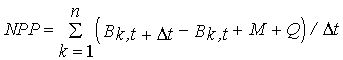(3)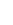NPP - Net primary productivity (g m-2 day-1);

B - Biomass (g m-2) ;

M - Losses through mortality (g m-2);

Q - Biomass removed by frond breakage (g m-2);

k - Size class;

n - total number of classes - 4;

t - Number of days between two consecutive samples.

Annual productivity was estimated by summation of the products of daily estimates over the respective sampling periods during a year.

Mortality was calculated from the loss rates of tagged fronds between two consecutive samples. It was assumed that the fraction of biomass lost by mortality was equal to the fraction of individuals lost from the population, since no relationship was found between mortality and plant size. Tag loss was estimated by double tagging several thalli (Santos, 1993b).

Biomass loss through partial frond breakage was calculated in two steps:

1) An empirical relationship between frond length and daily breakage probability was obtained (Fig. 2);

2) An empirical relationship between frond length and daily length reduction by breakage was also obtained (Fig. 3).

(Figs. 2 and 3, near here)

These relationships were calculated from data collected by Santos (1993a) between August 1989 and September 1990 and by the authors between September 1990 and October 1991. The first relationship was used to compute the percentage of fronds that were broken daily between two consecutive samples in each class. The second relationship was used to calculate the average daily reduction in the length of the fronds that were broken. A allometric relationship was used to compute the weight from the length before and after breakage. The difference in both weights gives the biomass lost by each frond. The product of this biomass by the breakage probability and the density of each class gives the total biomass lost per day per class.The total lost during the period t is given by the product of the daily biomass loss by the number of days between two consecutive samples.

Modelling

Model general structure and conceptualization

The main model equations are shown in Table 1. All computations are carried out for each size-class. From the net gain or loss in biomass and the number of individuals in each class the average individual weight of the class is computed. From an allometric relationship (eq. 4) the size is calculated. Growth is computed as the difference between the newly calculated size and the previous size.

(Table 1, near here)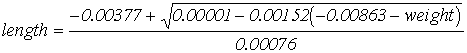(4)

(p < 0.001)

In the present case it is assumed that photosynthesis, respiration and exudation are independent of plant size. However, frond breakage is computed as a function of average class length as in the primary productivity computations (see above). Competition with other species is not considered. The comparison of the growth rates of tagged fronds with and without epiphytic algae did not show any significant differences (Duarte & Ferreira, 1993). Intraspecific competition seems to be reduced by the occurrence of storms and harvest, leading to major biomass losses (Santos, 1993a). Mortality is calculated as a constant fraction of the biomass and was obtained from the loss rate of tagged fronds. It is assumed that the population is in steady-state concerning the numerical density of the individuals: Therefore, dead fronds are replaced by class 1 recruits. Frond breakage is computed as described above. Photorespiration was not considered due to the absence of data on G. sesquipedale.

Parameter fitting

Gross productivity is expressed as a function of light intensity and temperature by a model described previously (Duarte, in press). Different parameters were used according to season or depth. These parameters were obtained by studying the relationship of productivity with light intensity and temperature on algae that were previously acclimated to different conditions. Four types of acclimation were employed, two simulating winter conditions and two different depths - approximately 9 and 13 m - and two simulating summer conditions and the same depths. Experimental evidence suggests that the model parameters are dependent on the recent history of the plants (Duarte & Ferreira, 1995). The equations for productivity calculation and respective parameters are shown in Table 2. At every time step the equation that was obtained with algae acclimated to conditions closest to the simulated ones was chosen. Equations obtained with algae from the winter acclimation were employed for winter and autumn simulations. Equations obtained with algae from the summer acclimation were employed for summer and spring simulations. Interpolation between the results obtained with different equations was carried out whenever the simulated conditions were within the range of the acclimation depths. A similar approach was used for respiration although this process was expressed solely as a function of temperature and season (Table 2). The conversion of productivity and respiration expressed in carbon units to biomass units was based on the carbon content of the studied species.

(Table 2, near here)

Exudation rate was also measured during the above mentioned experiments. Slightly different values were obtained with algae from the different acclimations. It was not possible to express exudation as a function of any environmental variable so it was computed as a rate that changed only with season or depth. The appropriate value was chosen following the same criteria used in the choice of equations for productivity calculation.

Size class structure and transitions

The usage of any kind of categories in mathematical modelling, such as size classes or compartments, usually implies that all individuals within a size class are assumed to be of the same size. In the Leslie matrix models the transitions between the classes occur in a progressive way, since the transition probabilities are usually less than one. The usual exceptions to this rule are the transitions to class 1 because each individual belonging to the larger size classes may produce more than one recruit.

In the present model the growth in each size class is computed from the net gains in weight. If it is assumed that all individuals are of the same size, the transition from one class to another occurs at the same time for all individuals of the departure class. This discrete type of transition may be acceptable when the size classes cover a very narrow range of sizes, but loses realism when the size classes are wider. Discrete transitions can lead to unreliable results when one or more size classes are emptied of individuals and the size distribution of the population becomes discontinuous, since in natural conditions this does not usually happen. Such transitions also tend to increase model instability. A possible solution to this problem is the usage of a statistical distribution of size within each class. In this case the growth of the individuals can be used to estimate their transition probabilities to a neighbouring size class.

In the present work the size distribution of individuals within each class was assumed to be gaussian. This assumption is a simplification, as well as the usual assumption of homogeneity. Its test against field data gave controversial results. In some cases the assumption seemed to be a good approximation whereas in other cases it was not validated. In fact, in a algal population where age-classes are not possible to define (as in the present case) there is no reason for this assumption to be always valid. But any assumption regarding intra-class structure will suffer from some disadvantages, specially when size-classes are considered.

To define a normal distribution two parameters are needed; the mean and the standard deviation. In the present case the mean is the current size of each class computed with the model at every time step. The standard deviation is unknown, but a value can be obtained to force the distribution to "stay" within the class limits. The values 2.5 were assumed to be the "limits" of the normal distribution. These values include more than 99% of the area under the standard normal curve, and were used as the standardised limits for the size classes. The standard deviation is computed as the minimum of the following equations:(5)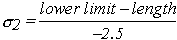(6)

The transitions of individuals may occur both to larger and smaller classes. In the first case, the transition will tend to decrease the average size of the destination class, whereas in the second case the opposite is true. The transition to smaller classes may occur through the breakage of the fronds, being more important when net productivity is negative. By knowing the mean size, its standard deviation and growth it is possible to calculate a critical standardised value above which the individuals should be transferred to another class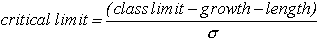(7)

The class limit to consider is the upper one if growth is positive and the lower one if growth is negative.

In Fig. 4 the effect of growth on the shape of the distribution is shown. The distribution shifts to the right and becomes narrower. All individuals above the critical limit are transferred to the neighbouring size class. The area of the curve to the right of the critical limit is calculated by integration. In the present work the formula of Hastings was used. It is important to remark that during the mentioned transitions biomass is conserved.

(Fig. 4, near here)

A total of 12 classes was used in model calculations: <5, 5-6, 6-7, 7-8, 8-9, 9-10, 10-11, 11-12, 12-13, 13-14 and >15 cm. However, for output only 4 classes were considered: <5, 5-10, 10-15 and >15 cm. Only the upper limit is included in each of the classes considered. The greater the number of classes used in model calculations the more reasonable is the assumption of homogeneity within the classes.

To preserve model stability, asymptotic biomass values were assumed for each class. These values were chosen from the maximal biomass observed in Cape Espichel (over 500 g (DW) m-2). When the biomass of a class is greater than its asymptotic value, the excess biomass and corresponding individuals are transferred randomly to the smaller classes or to the next class, simulating frond breakage or growth. If the excess occurs in class 1 then biomass is transferred to class 2. If the excess occurs in the last class, all biomass is transferred to smaller classes.

Model forcing functions

Daily water temperatures and global surface radiation obtained in two meteorological stations located near the study area were used as forcing functions for the model. Tidal height was simulated as described in Ferreira & Ramos (1989). Underwater light intensity was computed as described in Duarte & Ferreira (1993).

Nutrients were not used as forcing functions to the model. The capacity that some macroalgae have for luxury consumption makes the description of nutrient limitation more complicated. This capacity has been demonstrated for Gelidium species by Fredriksen & Rueness (1989). In the present case, dissolved nutrient data exists, but the available results do not allow the description of nutrient limitation.

Software development

Implementation of the model was carried out using object-oriented programming integrated in a existing model structure called EcoWin (Ferreira, 1995). An object named Gelididium sesquipedale was developed containing all attributes (variables) and methods (functions) used in the model calculations. A "man" object was also developed to simulate the harvest of the studied population. By coupling/uncoupling this object the impact of harvest on the studied population can be assessed. Different harvesting strategies can be simulated by simply changing the initialisation of some model conditions.

RESULTS AND DISCUSSION

G. sesquipedale population dynamics and productivity

Frond breakage probability and frond length reduction by breakage increase with frond initial size (Figs 2 and 3).  The larger fronds break easier than the smaller ones and the reduction in size is greater in the former than in the latter. These results can be explained by the fact that the larger fronds suffer greater drag induced by waves and currents (Koehl, 1986; Denny, 1988). In fact, frond breakage (Fig. 5) seems to be more related to frond size than to any seasonal factors, since the largest breaking ratios were observed in summer months when average frond size is higher (see above) and wave stress is lower. Wave height rarely exceeds 2 m during summer, whilst in winter values above 3 and 4 m are frequent in the west coast of Portugal.

(Fig. 5, near here)

In Fig. 6 frond mortality is shown, including the results obtained by Santos (1993a). The correlation between frond mortality and frond length is not significant (p > 0.05). Mortality does not seem to follow a distinct pattern throughout the year. The highest values were observed between August and September 1990 and between June and July 1991. The first maximum could be related to harvest in the study area (Santos, 1993a). The second maximum could be related to a worsening of sea conditions.

(Fig. 6, near here)

The biomass density dynamics of the studied species is shown in Fig. 7, together with the model results. Maximal values were observed during summer whilst minimal values occurred in winter. Similar results were obtained by Santos (1993a) in the same study area and by Juanes & Borja (1991) in the North of Spain.

(Fig. 7, near here)

The biomass dynamics of the four classes considered is shown in Fig. 8. The algae belonging to the intermediate sizes (classes 2 and 3) are dominant in terms of biomass. The larger fronds tend to increase their importance from spring to summer. This is also confirmed by Santos (1993a). During autumn and winter the biomass decreases due to mechanical stresses and metabolic losses affects all classes. However, the smaller classes are less affected because they receive a biomass input from the larger classes due to the breakage of the larger fronds.

(Fig. 8, near here)

Net primary productivity calculated by the method described in this work is shown in Table 3. The losses through mortality and frond breakage were larger than the net productivity. The reason for this result is that during the period used for productivity computation (May 1990 - May 1991) a net loss of biomass occurred. The average daily net primary productivity between sampling periods is shown in Fig. 9. The estimated values range between -2 and approximately 6 g (DW) m-2 day-1. Negative values occur mainly in autumn and winter months.

(Fig. 9 and Table 3, near here)

Model results

Standard simulation

Further parameter optimisation for the standard simulation was carried out after model calibration. The water extinction coefficient was the only parameter used in this final optimisation. Its value was set equal to 0.22, well within the range of the observed values (0.26  0.09) in the study area for a period of over a year. Model results were integrated over a depth range from 8 to 10 m with respect to the tidal datum, including the range of depths at which biomass sampling and tagging experiments took place.

In Fig. 7 the model biomass predictions and the observed values for the period between May 1990 and October 1991 are shown. Almost all model results are within the confidence limits of the observed values. The correlation between the measured and the predicted values is 0.74 (p < 0.05).

In Table 3 the annual net primary productivity predicted by the model can be compared to the value calculated by the method based on biomass differences, mortality and breakage ratios. The model prediction is only 15 % higher than the values estimated with this method.

In Fig. 10 the model biomass prediction is shown for a period of about five years, showing that after the second year of simulation the model remains stable. In Fig. 11 the model biomass prediction for each of the four classes is also shown for the same time interval. After the second year of simulation the population structure follows a pattern similar to that observed in Fig. 8, except that the biomass density of classes 1 and 4 is slightly higher than expected from field results.

(Fig. 11, near here)

In Fig. 12 the daily gross and net productivity predicted by the model are shown. Net primary productivity values obtained with the model are well within those estimated by the method described in this work. In autumn and winter net primary productivity is predominantly negative. These results can be explained by the low light intensities and temperatures during those seasons (Fig. 13) and were predicted earlier by the authors from laboratory data (Duarte & Ferreira, 1995). Considering that most of the G. sesquipedale stands on the west coast of Portugal are found at greater depths than those simulated (8 - 10 m), the negative net productivity during these seasons is probably more a rule than an exception.

(Fig. 12 and 13, near here)

The model results reported in Table 3 suggest that the greatest part of the biomass loss is attributable to mortality and frond breakage. The losses due to respiration and exudation are of the same order of magnitude. Since mortality and frond breakage are part of the net primary productivity, the negative values of this parameter are explained solely by the respiration and exudation losses. As suggested earlier (Duarte & Ferreira, 1995), the lower photosynthetic efficiencies of the winter acclimated plants, together with the lower light intensities and shorter photoperiods occurring in winter and autumn, explain the negative net productivity.

Sensitivity analysis

The sensitivity of the model was tested against some of the model initial conditions, forcing functions and parameters.

Sensitivity to the initial conditions

Concerning the initial conditions, the model sensitivity to the initial biomass levels was investigated, in order to evaluate the importance of the usually low precision of the biomass estimates on model predictions. As initial biomass input to the model both the upper and the lower confidence limits (at the 95 % confidence level) of the mean biomass observed in May 1990 (161.4 and 71.3 g (DW) m-2, respectively) were tested. This corresponds to a change of 39 % in relation to the average biomass - 116.3 g (DW) m-2. The larger biomass led to a net productivity prediction 38 % higher than under the standard simulation. The lower biomass led to a net productivity 37 % lower than under the standard simulation (Table 4).

Sensitivity to the model forcing functions

Concerning the model forcing functions, the sensitivity of the model to changes in the water light extinction coefficient and to temperature was tested. A 10% increase in the extinction coefficient led to a annual net productivity prediction of 104.0 g m-2 year-1, which is 45 % lower than the result obtained under the standard simulation (Table 4). As before, during autumn and winter net primary productivity is predominantly negative (not shown). A 10% decrease in the extinction coefficient was also tested. In this case a 61 % increase of the predicted primary productivity in relation to the standard simulation was obtained (Table 4).

From the above results it follows that the model is more sensitive to the light extinction coefficient than to the initial biomass conditions. The greater sensitivity of the net as compared to the gross primary productivity to the extinction coefficient are explained by the lower values of the latter. If gross productivity is affected by a change, the corresponding change in net productivity (which is the difference between gross productivity and the loss processes of respiration and exudation) will tend to be higher unless there is a similar change in respiration and exudation.

The sensitivity to temperature was investigated by assuming an average Summer temperature equal to the average Winter-Spring values (15 ºC). The predicted reduction in productivity (Table 4) demonstrates the potential for temperature limitation, since values close to 15 ºC can sometimes be observed during Summer (Fig. 13). In this case net productivity is less affected than gross productivity because the reduction in the latter during Summer is accompanied by a reduction in the respiration rates due to the lower temperatures.

Sensitivity to the model parameters

The sensitivity to mortality and breakage ratios were investigated by changing the default values to 10 %. From the results shown in Table 4 it is clear that the model is more sensitive to changes in mortality than to changes in the breakage ratio which in fact are almost irrelevant in the model output. The model sensitivity to changes in mortality is also low. Since both mortality and the breakage ratios are very difficult to estimate and subject to many errors the low model sensitivity to them is an important result. The higher sensitivity of gross rather than net productivity to changes in mortality is explained by the fact that the changes in gross productivity at the population level reflected by the changes in the biomass standing stock resultant from different mortalities, are accompanied by changes in the population respiration rates, which tend to compensate for changes in net productivity.

The sensitivity of the model to the usage of only one set of parameters in the productivity equation was also investigated (shown in eq. 8). The parameters were estimated by analysing together the four data sets used in the parameter estimation of equations 12 to 15 (see Duarte & Ferreira, 1995). As can be seen in Table 4 the predicted annual productivity estimates are much lower (-14.1 and -17.9 % for annual gross and net productivity, respectively) than those obtained under the standard simulation. The nonlinearities between productivity and the forcing functions light and temperature may explain this result which highlights the importance of considering seasonal adaptation on primary productivity simulation.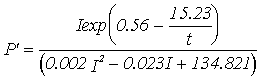(8)

A similar approach was followed concerning respiration (eq. 9). In this case the differences relative to the standard simulation are smaller (Table 4). This may be partly explained by the fact that the annual losses by respiration are much lower than the annual net productivity (Table 3).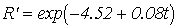(9)

(Table 4, near here)

Harvest simulation

Harvest usually takes place from the end of July to the end of the year. In the following simulations it was assumed that the plants were cut instead of plucked from the rocks, since the disadvantages of this harvesting technique have already been discussed and demonstrated  (Salinas et al., 1976; Santelices, 1988; Santos, 1993c). There are already cutting devices operated from boats that allow the cutting height to be chosen and do not decrease the plant density (Santos, 1993c). It was assumed that cutting occurred only once in a hypothetical 1 m2 area. The recovery of the population was then followed. The initial conditions were those of the standard simulation after allowing the model to reach stability.

In the first of these harvest simulations a cutting height of 3 cm was assumed, with harvest taking place at the end of July, leaving all harvested plants with a length of 3 cm after cutting. In Fig. 14 the predicted results are shown. After harvest, the remaining biomass was approximately 13 % of the initial value. Three years later, the recovery of the population was complete. The total harvested biomass was 167 g (DW) m-2. All individuals entered into class 1 after cutting. The recruitment to class 2 occurred in the first year after the harvest, whilst the recruitment to classes 3 and 4 occurred only in the second year (Fig. 14). Since the lower dimensional limit of class 4 is 15 cm, it follows that the algae that recruited to this class grew approximately 6 cm year-1 after harvest. The growth rates referred by Santos (1994) point to an annual maximum of 8 - 9 cm. However, these results were obtained by considering only the tagged algae that grew between two measurements, so they correspond to a potential maximal growth rate that is hardly ever attained.

(Fig. 14, near here)

Further simulations were carried out assuming cutting heights of 5, 7 and 9 cm. The results obtained are synthesised in Table 5, where the average annual yield is plotted against the time for the population to recover after cutting. It was assumed that cutting is not repeated until full recovery of the population. A cutting height smaller then 7 cm may lead to a regrowth period of two years or more. By keeping the cutting height around 7 cm it is possible to harvest every year and to obtain an average yield larger then cutting to a lesser height every two or three years. These results demonstrate the potential usefulness of the model and suggest some interesting options to increase harvest yield.

(Table 5, near here)

The results obtained confirm that during autumn and winter the primary productivity of an important part of the G. sesquipedale stands is negative. During these periods major biomass losses occur because of storms and metabolic losses. This natural "harvest" may be anticipated by an industrial harvest, that may be kept at a sustainable yield by appropriately choosing the cutting heights and harvesting frequency.

CONCLUSIONS

The main objective of this model was to simulate simultaneously the frond individual weight/size, the biomass and density changes of an algal population divided in size classes. The simulation of the frond size is important when the population is to be harvested and the optimal cutting height is to be defined. Besides that, only by simulating size is it possible to describe some demographic aspects and control some model results in terms of their quality. For example, it is important to calibrate the model in order that the size of the plants does not exceed a maximal value, determined from field data. When this value is reached the breakage ratio should be reformulated in order to avoid any further growth.

The usage of size classes has important implications in model calibration and validation, since the distribution of biomass among the classes follows certain patterns that must be taken into account during the modelling process. If classes are not considered and biomasses treated as a single class the predicted values are much higher than when classes are considered and asymptotic biomass values (see Method's section) are defined for each class. The rationale behind the use of these asymptotic values is related to those processes that are either not explicitly or poorly simulated by the model, such as space limitation and frond breakage ratios.

The assumption that the distribution of sizes within the classes follows the normal distribution and the usage of the algorithm described previously, allowed the simulation of the biomass transfer between classes in a quasi-continuous way. It is likely that the methods described here concerning between-class transfer can be employed in the simulation of other plant and animal populations. The assumption of normality is debatable, but the usual assumption of size/weight homogeneity within the classes is also arguable and leads to unreliable delays in the between-class transfer. These problems can be partially solved only by considering a very large number of classes which are reflected in more computing time. In the case of animal or plant populations in which cohorts are easy to define, the assumption of normality in size/weight distributions within the classes is less questionable since it is clear that these distributions are close to normal.

Further improvements of the present model include the dynamic description of photosynthesis in a way similar to that discussed by Pahl-Wostl & Imboden (1990). The equations used in the present work do not take into account the developing time of photoinhibition. This may be done by describing the first parameter (responsible for the photoinhibited behaviour) of the denominator of equations 14-17 as a function of the time the plants are exposed to a light level above the optimum. The authors' results (unpublished) suggest that under certain conditions the predicted primary productivity may be much higher than expected by considering this time dependency. However, the available data does not allow an accurate estimation of its importance for the studied species.

Another important field of research is the seasonal adaptation of the photosynthetic and respiratory responses to light and temperature. The usage of different equations according to time of the year or depth as in the present work, is far from optimal. However, it was the only available way to include some of the variability for these responses.

It is also important to consider the time variability of the allometric relationships used to calculate growth from biomass changes and vice-versa. These relationships are expected to change over time because, among other things, of the endogenous annual cycles (Lüning, 1993) demonstrated for some algal species. The growth in weight may not always coincide with the growth in length.

The usage of an object oriented programming approach allows the fast development of descendants to any general object inheriting their attributes and methods (Silvert, 1993; Ferreira, 1995). In the present case descendants to the G. sesquipedale object may be developed as more information becomes available concerning any of the topics just discussed. The programming work would consist only of adding the new functions, or overriding the old ones. The extension of this model to other species is likewise straightforward.

Acknowledgements

This work was supported by JNICT (PhD grant Nº154 - IG) and INETI. The authors wish to thank C. Peneda for support of this research, and L. Saldanha, L. Fonseca and O. Luis (LMG-IMAR) and F. Duarte (INETI), for making available laboratory facilities. The authors also thank R. Santos for making available part of his data and A. Afonso for his help on statistics.

REFERENCES

Ang, P.O. Jr, 1987. Use of projection matrix models in the assessment of harvesting strategies for Sargassum. Hydrobiologia, 151/152: 335-339.

Ang, P.O. Jr and De Wreede, R.E., 1990. Matrix models for algal life history stages. Mar. Ecol. Prog. Ser., 59: 171-181.

Brinkhuis, B.H., Tempel, N.R. and Jones, R.F., 1976. Photosynthesis and respiration of exposed salt-marsh fucoids. Marine Biology, 34: 349 - 359 .

Caswell, H., 1986. Life cycle models for plants. In: Lectures on mathematics in the life sciences, Vol. 18, pp. 171-223. Ed. by the American Mathematical Society.

Chapman, A.R.O., 1993. 'Hard' data for matrix modelling of Laminaria digitata (Laminariales, Phaeophyta) populations. Hydrobiologia, 260/261: 263-267.

Dixon, P.S., 1973. Biology of the Rhodophyta. Oliver & Boyd, Edinburgh.

Denny, M.W., 1988. Biology and the mechanics of the wave-swept environment. Princeton University Press, Princeton.

Duarte, P., 1995. A mechanistic model of the effects of light and temperature on algal primary productivity. Ecol. Modelling, 82: 151-160.

Duarte, P., and Ferreira, J.G., 1993. A methodology for parameter estimation in seaweed productivity modelling. Hydrobiologia, 260/261: 183-189.

Duarte, P. and Ferreira, J.G., 1995. Seasonal adaptation and short-term metabolic responses of Gelidium esquipedale to varying light and temperature. Mar. Ecol. Prog. Ser, 121: 289-300.

Eilers, P.H.C. and Peeters, J.C.H., 1988. A model for the relationship between light  intensity and the rate of photosynthesis in phytoplankton. Ecol. Modelling, 42: 199 - 215 .

Ferreira, J.G. and Ramos, L., 1989. A model for the estimation of annual production rates of macrophyte algae. Aquatic Botany, 33: 53-70.

Ferreira, J.G., 1995. ECOWIN - An object-oriented ecological model for aquatic ecosystems. Ecol. Modelling, 79: 21-34.

Fredriksen, S. and Rueness, J., 1989. Culture studies of Gelidium latifolium (Grev.) Born et Thur. (Rhodophyta) from Norway.Growth and nitrogen storage in response to varying photon flux density, temperature and nitrogen availability. Botanica Marina, 32: 539 - 546.

Iwakuma, T. and Yasuno, M., 1983. A comparison of several mathematical equations describing photosynthesis-light curve for natural phytoplankton populations. Arch. Hydrobiol., 97: 208-226.

Jassby, A.D. and Platt, T., 1976. Mathematical formulation of the relationship between photosynthesis and light for phytoplankton. Limnol. Oceanogr., 21: 540-547.

Keller, A.A., 1989. Modeling the effects of temperature, light, and nutrients on primary productivity: An empirical and a mechanistic approach compared. Limnol. Oceanogr., 34: 82-95.

Koehl, M.A.R., 1986. Seaweeds in moving water: form and mechanical function. In: Givnish, T.J. (ed.) On the economy of plant form and function, Cambridge University Press, Cambridge, pp. 603-634.

Kremer, B.P., 1981. Aspects of carbon metabolism in marine macroalgae. Oceanogr. Mar. Biol. Ann. Rev., 19: 41-94.

Leslie, P.H., 1945. On the use of matrices in certain population mathematics. Biometrica, 33: 183-212.

Leslie, P.H., 1945. Some further notes on the use of matrices in population mathematics. Biometrica, 35: 213-245.

Lüning, K., 1993. Environmental and internal control of seasonal growth in seaweeds. Hydrobiologia, 260/261: 1-14.

Margalef, R., 1977. Ecologia. Omega, Barcelona.

Odum, H.T., 1983. Systems ecology: An introduction. John Wiley & Sons, Inc., Toronto.

Pahl-Wostl, C and Imboden, DM, 1990. DYPHORA - a dynamic model for the rate of photosynthesis of algae. J. Plankton Res., 12: 1207-1221.

Platt, T., Gallegos, C.L. and Harrison, W.G., 1980. Photoinhibition of photosynthesis in natural assemblages of marine phytoplankton. J. Mar. Res., 38: 687-701.

Salinas, J.M., Ramirez, B.R. and Olivet, R.G., 1976. Biometría en Gelidium sesquipedale (Rhodophyta). Bol. Inst. esp. Oceano., 225: 127-166.

Santelices, B., 1988. Synopsis of biological data on the seaweed genera Gelidium and Pterocladia (Rhodophyta). FAO Fisheries Synopsis, 145.

Santos, R. and Duarte, P., 1991. Marine plant harvest in Portugal. Journal of Applied Phycology,  3: 11-18.

Santos, R., 1993a. Plucking or cutting Gelidium sesquipedale? A demographic simulation of harvest impact using a population projection matrix model. Hydrobiologia, 260/261: 269-276 .

Santos, R., 1993b. Population biology of the commercial seaweed, Gelidium sesquipedale: Biological input for resource management. PhD Thesis, Dalhouise University, Halifax, Nova Scotia, Canada.

Santos, R.  1993c. A multivariate study of biotic and abiotic relationships in a subtidal algal stand. Mar. Ecol. Prog. Ser., 94: 181-190.

Santos, R., 1994. Frond dynamics of the commercial seaweed Gelidium sesquipedale: effects of size and frond history. Mar. Ecol. Prog. Ser., 107: 295-305.

Seip, K.L., Lunde, G., Melsom, S., Mehlum, E., Melhuus, A. and Seip, H.M., 1979. A mathematical model for the distribution and abundance of benthic algae in a norwegian fjord. Ecol. Modelling, 6: 133-166.

Seip, K.L., 1980a. A computational model for growth and harvesting of the marine algae Ascophyllum nodosum. Ecol. Modelling, 8: 189-199.

Seip, K.L., 1980b. A mathematical model of competition and colonisation in a community of marine benthic algae. Ecol. Modelling, 10: 77-104.

Silvert, W., 1993. Object-oriented ecosystem modelling. Ecol. Modelling, 68: 91-118.

Sokal, R.R. and Rohlf, F.J., 1981. Biometry. The principles and practice of statistics in biological research. W.H. Freeman & Co, San Francisco.

Steele, J.H., 1962. Environmental control of photosynthesis in the sea. Limnol. Oceanogr., 7: 137 - 150.

Usher, M.B., 1966. A matrix approach to the management of renewable resources, with special reference to selection forests. J. Appl. Ecol., 3: 355-367.

Wiegert, R.G. and Evans, F.C., 1964. Primary production and disappearance of dead vegetation on an oil field in south-eastern Michigan. Ecology, 45: 49-63.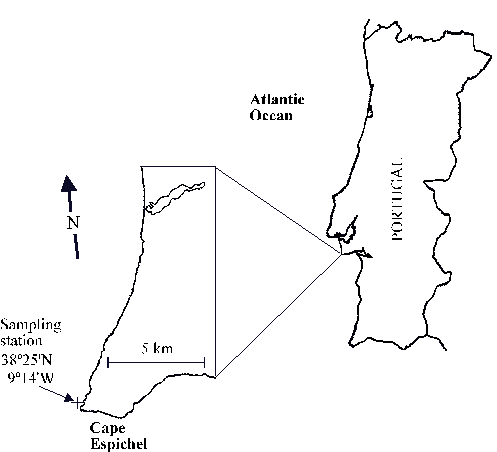Fig. 1 - Study area.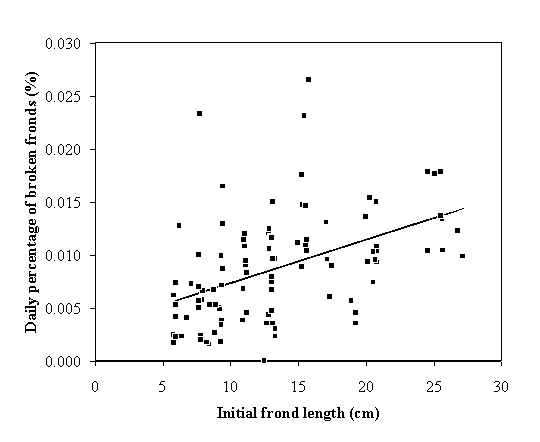Fig. 2 - Average daily percentage of broken fronds versus initial frond length. The regression line (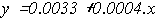) is significant (p < 0.05).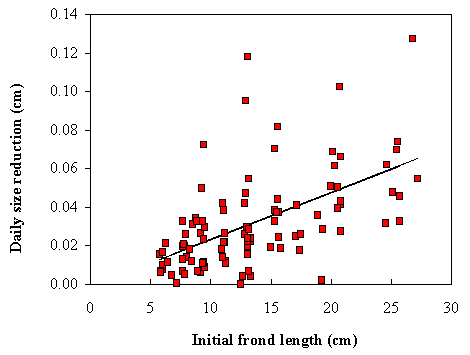Fig. 3 - Size reduction versus initial frond length. The regression line (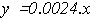) is significant (p < 0.001).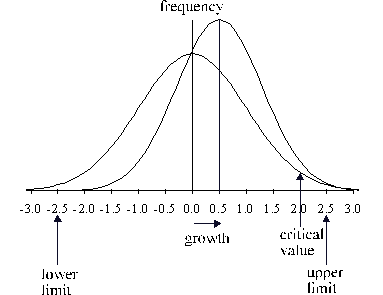Fig. 4 - The effect of growth on the shape of the size distribution of individuals within the classes calculated by the model. The curve shifts to the right after growth is computed. The area to the right of the critical value corresponds to the transition probability (see text).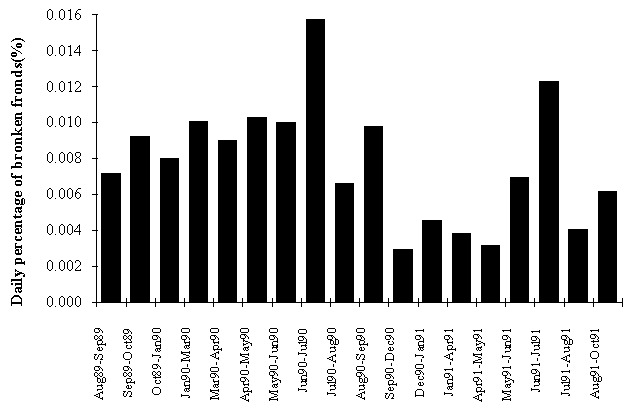Fig. 5 - Frond daily breakage (% of tagged fronds).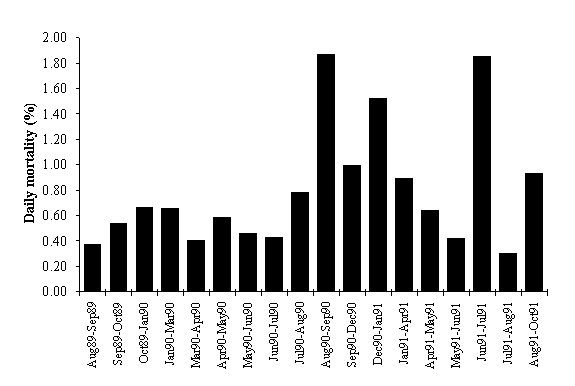Fig. 6 - Daily mortality (% of tagged fronds).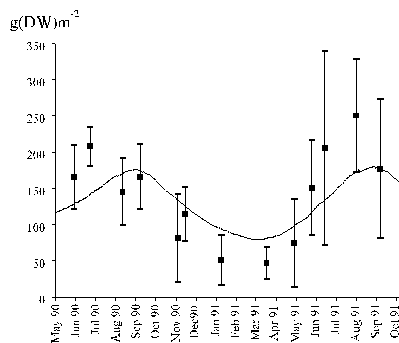Fig. 7 - Biomass density dynamics. Model results of the standard simulation (line) and observed values (squares). The vertical bars are 95% confidence limits.

g(DW)m-2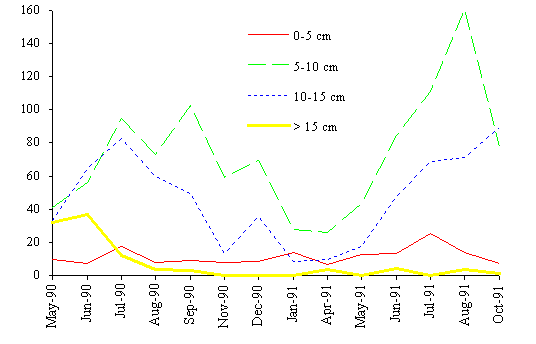Fig. 8 - Biomass density dynamics of the four classes considered.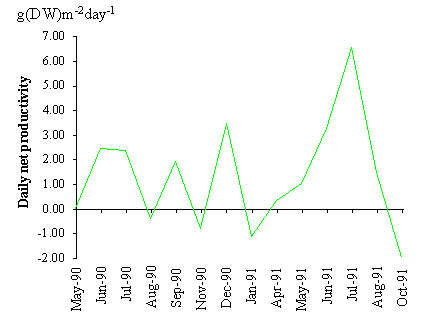Fig. 9 - Daily net productivity estimated by the method described in this work, from biomass changes and losses due to mortality and breakage.

g(DW)m-2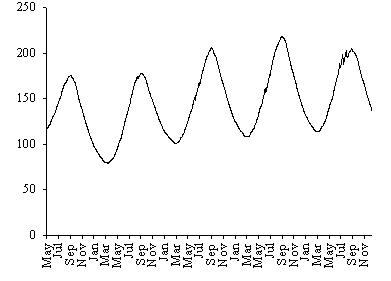Fig. 10 - Standard simulation. Biomass density predicted by the model.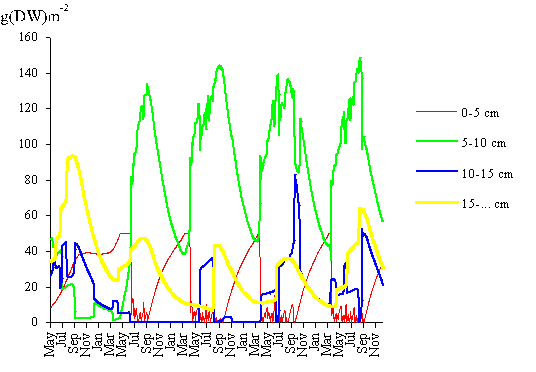Fig. 11 - Standard simulation. Biomass density variation of the four classes considered predicted by the model (see text).

g(DW)m-2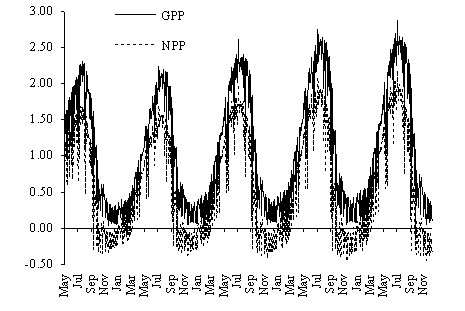Fig. 12 - Standard simulation. Gross and net daily primary productivity (GPP and NPP, respectively) predicted by the model.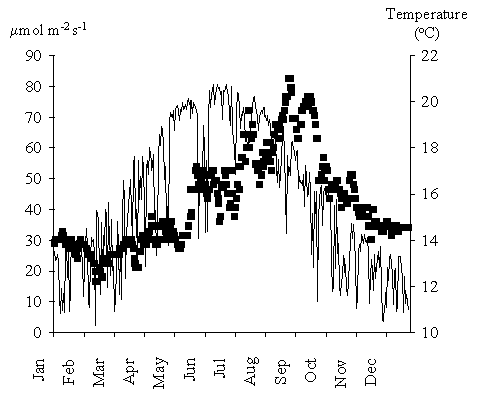Fig. 13 - Daily average light intensity (line) at 9 m depth (reported to tidal datum) and temperature (squares). The light values were computed from available time series and assuming a water light extinction coefficient of 0.22.

###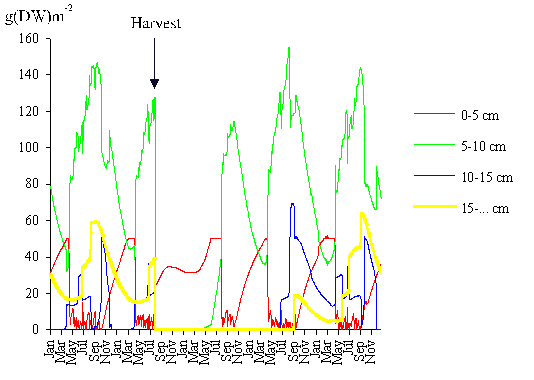Fig. 14 -  Biomass density variation of the four classes considered predicted by the model, before and after cutting the population to a height of 3 cm at the end of July. All other conditions as in the standard simulation (see text).

Table 1  - Main model equations.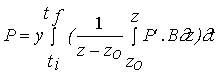(10)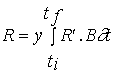(11)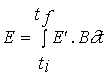(12)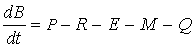(13) B - Biomass (g m-2) z e z0- Integration depths (m) T*= tf - ti- Model timestep P' - Gross productivity(g C g-1 h-1)** R' - Respiration rate (g C g-1 h-1)*** P - Gross productivity (g m-2 T*-1) R -Respiration rate (g m-2 T*-1) E' - Exudation rate(g g-1 h-1) E - Exudation rate (g m-2 T*-1) Q - Frond breakage rate (g m-2 T*-1) M - Mortality (g m-2 T*-1) y - Conversion factor between carbon and dry weight * 0.2 days. ** Computed as a function of light intensity and temperature. *** Computed as a function of temperature.

Table 2 - Equations for gross productivity and respiration; P' - Gross productivity (g C g-1 h-1), R' - Respiration rate (g C g-1 h-1***), I - light intensity (mol photons m-2 s-1), t (ºC) (see text).

 Acclimation Equations Winter 13 m depth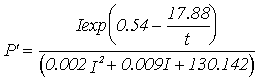(14) Winter 9 m depth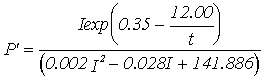(15) Summer 13 m depth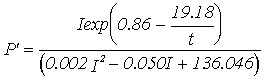(16) Summer 9 m depth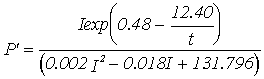(17) Winter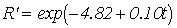(18) Summer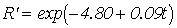(19)

Table 3 - Annual productivity and biomass loss estimates obtained by the method described in this work and by the model. GPP and NPP - Gross and net primary productivity, respectively; POM - Losses through particulate organic matter from frond mortality and frond breakage; DOM - Losses through dissolved organic matter (exudation) and R - Respiration. All values in g (DW) m-2year-1.

 GPP NPP GPP/ Biomass POM DOM R Biomass differences and biomass removal estimates 162.5 205.2 Model results 339.3 187.7 2.9 210.0 51.1 100.6

Table 4 - Sensitivity analysis results (see text).

 GPP NPP Calculated value (gDWm-2year-1) Relative change (%) Calculated value (gDWm-2year-1) Relative change (%) Standard simulation 339.1 - 187.5 - 10% Increase in the initial biomass 456.6 +34.7 257.8 +37.5 10% Decrease in the initial biomass 214.0 -36.9 119.1 -36.5 10% Increase in the ext. coef. 221.1 -34.8 104.0 -44.5 10% Decrease in the ext.coef. 481.7 +42.1 301.6 +60.9 Using in Summer the average Winter-Spring temperature 297.8 -12.2 160.6 -14.3 10% Increase in mortality 329.8 -2.7 185.6 -1.0 10% Decrease in mortality 367.5 +8.4 202.4 +7.9 10% Increase in frond breakage 338.9 -0.1 187.4 -0.1 10% Decrease in frond breakage 339.2 0.0 187.6 +0.1 Only one set of parameters in the prod. eq. 291.4 -14.1 154.0 -17.9 Only one set of parameters in the resp. eq. 338.3 -0.2 180.2 -3.9

Table 5 - Mean annual yield as a function of regrowth time assuming average cutting heights of 3, 5, 7 and 9 cm respectively (see text).

 Cutting heigh (cm) Regrowth period (years) Mean annual yield (g(DW)m-2) 3 3 55.1 5 2 56.1 7 1 66.6 9 1 43.4